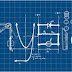Physics Quiz and Answers for LDC Exams: In this post we are sharing the previous year Physics Quizzes with answers asked in the previous year SSC Examination. Below we are given 15 objective multiple choice questions with answers asked in the Combined Higher Secondary Level Examination. This time SSC Recruitment Board has decided to conduct the written examination in Online Computer Based Test (CBT). These bits helpful for your preparation.

## Physics Quiz and Answers for LDC Exams

1. The SI of electric flux is?
A) Weber
B) Newton per coulomb
C) Voltmeter
D) Jule per coulomb
Answer: A

2. Three different capacitors are connected in series then?
A) They will have equal charges
B) They will have same potential
C) Both a and b
D) None of these
Answer: A

3. Equal charges are given to two spheres of different radii the potential will be?
A) More on smaller sphere
B) More on bigger sphere
C) Equal on both the sphere
D) None of these
Answer: A

4. In bringing an electron towards another electron the electrostatic potential energy of the system?
A) remains same
B) Becomes zero
C) Increases
D) Decreases
Answer: C

5. Capacity of a conductor depends upon?
A) Size of conductor
B) Thickness of conductor
C) Material of conductor
D) All of these
Answer: A

6. A point charge is brought in an electric field. The field at a nearby point?
A) May increase if the charge in positive
B) May decrease if the charge is negative
C) Both a band b
D) None of these
Answer: C

7. A steady current is flowing in a conductor of non-uniform cross-section.  The charge passing through any cross section per unit time is?
A) Directly proportional to the area of cross-section
B) Inversely proportional to the area of cross-section
C) Proportional to square of the area of cross-section
D) Independent of the area of cross-section
Answer: D

8. If the length of a wire is doubled an its cross section is also doubled, then its resistance will?
A) Become one fourth
B) Become four time
C) Become two times
D) Remain unchanged
Answer: D

9. The resistance of a wire is R. it is stretched uniformly so that its length is doubled. The resistance now becomes?
A) 2R
B) R/2
C) 4R
D) R/4
Answer: C

10. The specific resistance of a wire?
A) Varies with its length
B) Varies with its cross-section
C) Varies with its mass
D) Does not depends on its length, cross-section and mass
Answer: D

11. The reciprocal of specific resistance is?
A) Conductive resistance
B) Specific conductance
C) Resistance
D) Plate resistance
Answer: B

12. Kirchooff’s two laws for electrical circuits are manifestations of the conservation of?
A) Charge only
B) Momentum only
C) Energy only
D) Both energy and charge
Answer: D

13. A galvanometer is converted into an ammeter when we connect a?
A) High resistance in series
B) High resistance in parallel
C) Low resistance in series
D) Low resistance in parallel
Answer: D

14. A galvanometer is converted into a voltmeter when we connect a?
A) High resistance in series
B) High resistance in parallel
C) Low resistance in parallel
D) Low resistance in series
Answer: A

15. The reciprocal of resistance is?
A) Conductance
B) Resistivity
C) Conductivity
D) None of the above
Answer: A

### Related Articles for SSC CHSL Physics

Physics Quiz and Answers for LDC Exams SSC CHSL Physics Asked QuestionsReviewed by mani on 19:27:00 Rating: 5